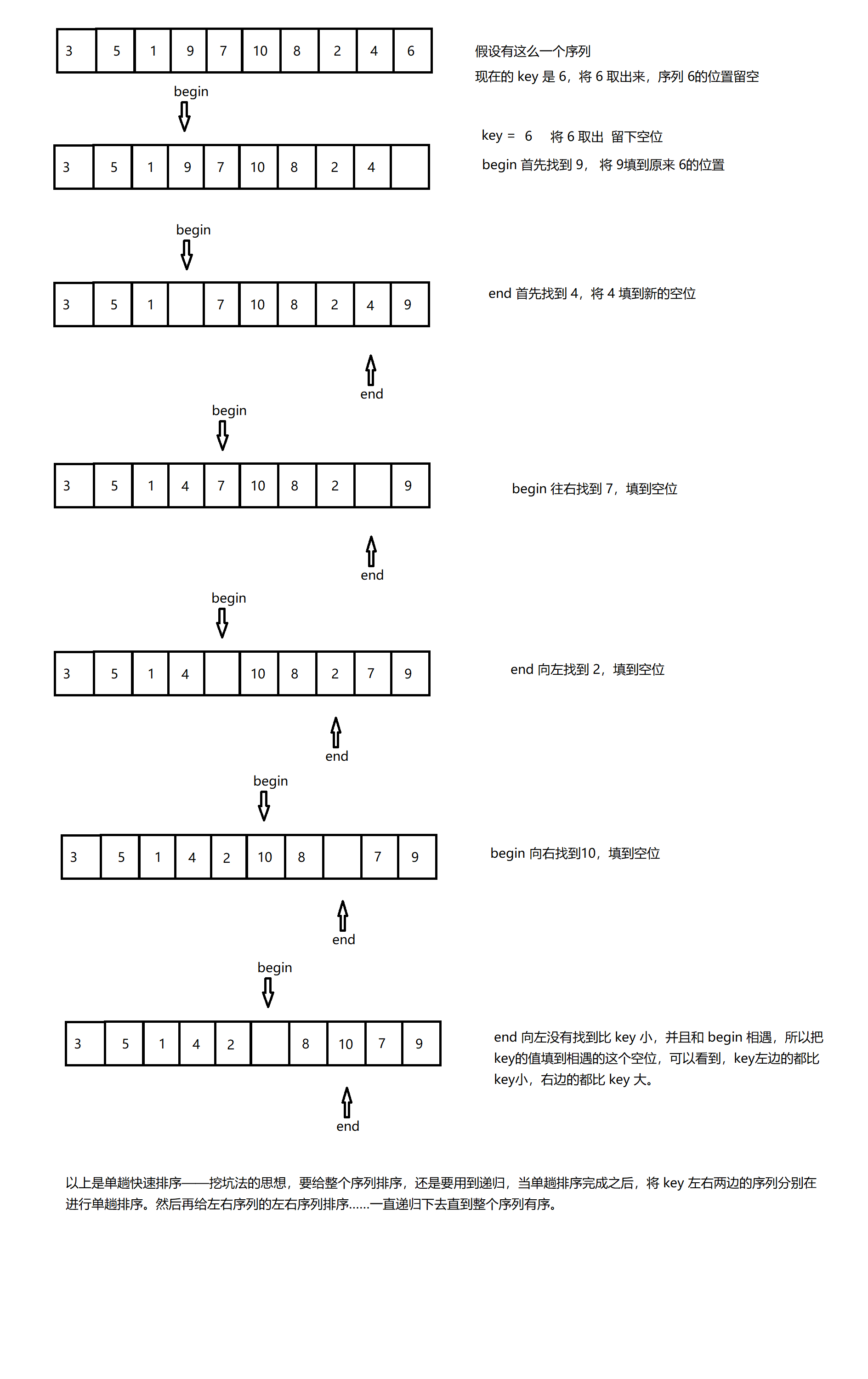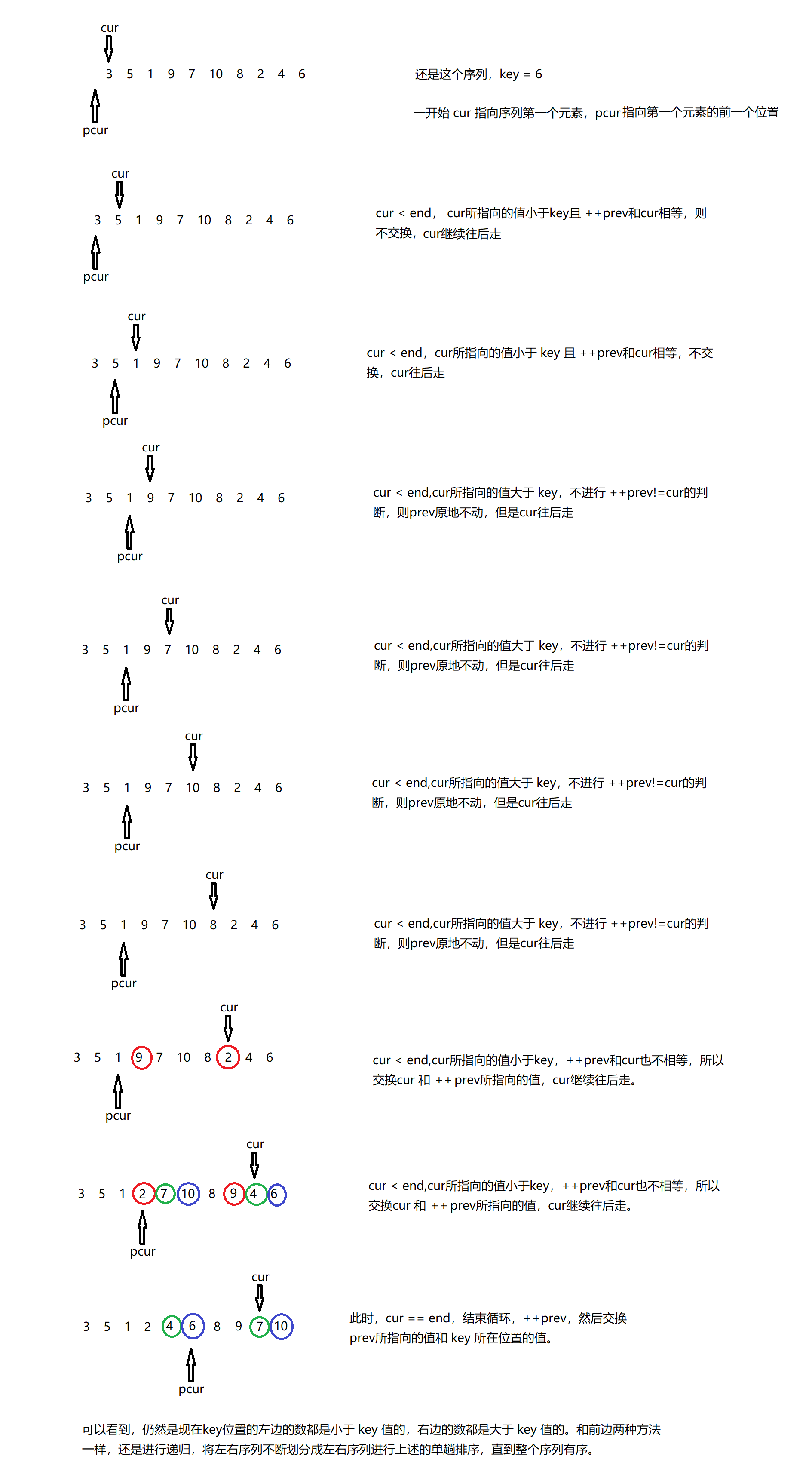# 快速排序

• 左右指针法
• 挖坑法
• 前后指针法

1. 首先在序列里边找出来 key 值，一般是用的序列最后一个元素作为 key 值。
2. 指针 begin 从序列左边开始往右边找比 key 值大的元素，若找到，停下，若没找到，继续往右走。
3. 指针 end 从序列右边开始往左边找比 key 值小的元素，若找到，停下，若没找到，继续往左走。
4. 重复上述 (2)(3) 步,直到begin 和 end 相遇，交换 begin 和 end 的值。
上述 4 个步骤结束之后，已经交换过的 key 值左边都是比 key 值小的元素，右边都是比 key 值大的元素。接着将 key 值左右两边的序列看做两个单独的序列。再次重复上述 4 个步骤。void Swap(int *x,int *y) {
int tmp;
tmp = *x;
*x = *y;
*y = tmp;
}

int PartSort(int *a, int begin, int end) {
int key = a[end];  // 选择序列最边边的值为 key
int keyindex = end;    // 或许 key 值的下标，因为最后是和 key 值所在的位置进行交换，不是和 key 值进行交换更改 key 值。
while (begin < end) {
// while 里边加判断 begin<end，避免begin 和 end 错过
while (begin < end && a[begin] <= key)
++begin;
while (begin < end && a[end] >= key)
--end;
Swap(&a[begin], &a[end]);
}
Swap(&a[begin], &a[keyindex]);
return begin;
}

void QuickSort(int *a, int begin, int end) {
//  begin>=end说明有序，有序就直接返回
if (begin >= end)
return;
int KeyIndex = PartSort(a, begin, end);
QuickSort(a, begin, KeyIndex - 1);
QuickSort(a, KeyIndex + 1, end);
}


1. 先选定一个 key 值，还是选择序列最右边的值吧。
2. 将 key 值所在的值拿出来，把 key 值所在的位置留个空。
3. 左边指针 begin 从序列左边往右边找比 key 值大的，如果没找到，继续往右走，找到了就停下。把此时 begin 所在位置的值填到 key 处的空白。
4. 右边指针 end 从序列的右边往左找比 key 值小的，如果没找到，继续往左走，找到了就停下。把此时 end 所在位置的值填到 begin 处的空白。
5. 左边指针 begin 继续往右走，找到比 key 大的把此处 begin 的值填到 end 处的空白。
6. 重复上述 (4)(5) 步，直到 begin 和 end 相遇，将 key 的值填到相遇处。void Swap(int *x,int *y) {
int tmp;
tmp = *x;
*x = *y;
*y = tmp;
}

int PartSort2(int *a,int begin,int end) {
int key = a[end];
while (begin < end) {
while (begin < end && a[begin] <= key)
++begin;
a[end] = a[begin];

while (begin < end && a[end] >= key)
--end;
a[begin] = a[end];
}
a[begin] = key;
return begin;
}

void QuickSort(int *a, int begin, int end) {
if (begin >= end)
return;
int KeyIndex = PartSort2(a, begin, end);
QuickSort(a, begin, KeyIndex - 1);
QuickSort(a, KeyIndex + 1, end);
}


1. 先定两个指针 cur 指向序列的第一个数，prev 指向 cur 的前一个位置。
2. 如果cur所指向的位置，小于 end 的位置，cur所指向的值小于key，并且++prev 和 cur 所指向位置不一样，交换cur 和 prev 所指向的值，cur 再往后走。如果cur 所指向的值小于 key，但是 ++prev 和 cur 所指向的位置一样，则不进行交换。但是 prev 向后走了一个位置，因为是前++。
3. 如果cur 所指向的值 大于key 的值，那么只 ++ cur，不 ++ prev。
4. 当 cur 所指向的位置和 prev 所指向的位置相同时，prev 向后走一个位置，然后和 key 所指向的值进行一个交换void Swap(int *x,int *y) {
int tmp;
tmp = *x;
*x = *y;
*y = tmp;
}

int PartSort3(int *a,int begin,int end) {
int prev = begin - 1;
int cur = begin;
int key = a[end];
while (cur < end) {
if (a[cur] < key && ++prev != cur)
Swap(&a[cur],&a[prev]);
++cur;
}
++prev;
Swap(&a[end],&a[prev]);
return prev;
}

void QuickSort(int *a, int begin, int end) {
if (begin >= end)
return;
int KeyIndex = PartSort3(a, begin, end);
QuickSort(a, begin, KeyIndex - 1);
QuickSort(a, KeyIndex + 1, end);
}©️2019 CSDN 皮肤主题: 大白 设计师: CSDN官方博客